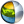Cg 3.1 Toolkit Documentation Cg / Standard Library / unpack

## Name

unpack - unpacks a single values into different formatted values

## Synopsis

```half2  unpack_2half(float a);
float2 unpack_2ushort(float a);
half4  unpack_4byte(float a);
half4  unpack_4ubyte(float a);
```

a
Value to unpack

## Description

unpack_2half unpacks a 32-bit integer value into two 16-bit floating point value.

unpack_2ushort unpacks two 16-bit unsigned integer values from a and scales the results into individual floating point values between 0.0 and 1.0.

unpack_4byte unpacks four 8-bit integers from a and scales the results into individual 16- bit floating point values between -(128/127) and +(127/127).

unpack_4ubyte unpacks the four 8-bit integers in a and scales the results into individual 16- bit floating point values between 0.0 and 1.0.

## Reference Implementation

unpack_2half could be implemented this way:

```half2  unpack_2half
{
result.x = (a >> 0) & 0xFF;
result.y = (a >> 16) & 0xFF;
return result;
}
```

unpack_2ushort could be implemented this way:

```float2  unpack_2ushort
{
float 2 result;
result.x = ((x >> 0) & 0xFFFF) / 65535.0;
result.y = ((x >> 16) & 0xFFFF) / 65535.0;
return result;
}
```

unpack_4byte could be implemented this way:

```half4  unpack_4byte(float a)
{
half4 result;
result.x = (((a >> 0) & 0xFF) - 128) / 127.0;
result.y = (((a >> 8) & 0xFF) - 128) / 127.0;
result.z = (((a >> 16) & 0xFF) - 128) / 127.0;
result.w = (((a >> 24) & 0xFF) - 128) / 127.0;
return result;
}
```

unpack_4ubyte could be implemented this way:

```half4  unpack_4ubyte(float a)
{
half4 result ;
result.x = ((a >> 0) & 0xFF) / 255.0;
result.y = ((a >> 8) & 0xFF) / 255.0;
result.z = ((a >> 16) & 0xFF) / 255.0;
result.w = ((a >> 24) & 0xFF) / 255.0;
return result;
}
```

## Profile Support

unpack is supported in fp30, fp40, gp4, gp5| Cg Toolkit | Download | Release Archive | Profiles | Reference | Books | Discussions |